## Binormal Vector(1)(2)

where the unit Tangent Vectorand unit principal'' Normal Vector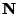are defined by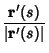(3)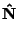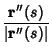(4)

Here,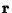is the Radius Vector,is the Arc Length,is the Torsion, and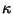is the Curvature. The binormal vector satisfies the remarkable identity(5)

Kreyszig, E. Binormal. Moving Trihedron of a Curve.'' §13 in Differential Geometry. New York: Dover, p. 36-37, 1991.